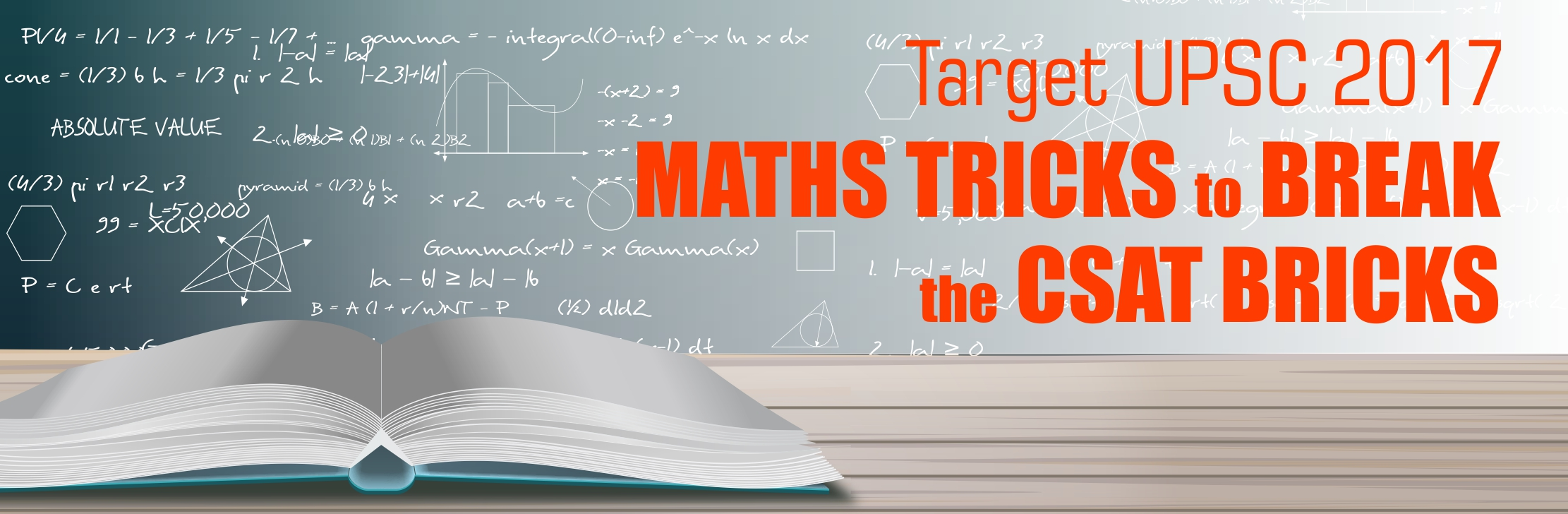# Target UPSC 2019 Maths Tricks to Break the CSAT BricksA good chunk of the UPSC civil services aspirants are from a non-technical background. A lot of the IAS candidates especially those having a humanities background fear the maths part in the CSAT paper. As you know, as per the new UPSC civil services exam pattern, the prelims consists of two papers:

1. Paper I: General Studies – 2 hours/200 marks – 100 questions
2. Paper II: Aptitude (also called Civil Services Aptitude Test or CSAT) – 2 hours/200 marks – 80 questions

Check syllabus for UPSC Prelims CSAT here.

At the outset, know that the mathematics part of the CSAT is of a basic level and you need not be scared of it. You only need to brush up on your high school mathematics and do some serious practice. With this, you should be able to clear the maths portion with ease despite your background. Remember, we’ve all had some maths background at the school level.

You have to refresh your school level algebra, numeracy, geometry, and trigonometry. Questions would be mostly on percentages, averages, age, time and work, time and distance, profit and loss, probability, etc.

Serious IAS aspirants cannot afford to ignore the maths portion in CSAT as this part is very scoring and with only a bit of work, you can do well. The CSAT paper is of a qualifying nature and unless you clear it by getting 33%, you will not be able to have a go at the UPSC mains. No matter how hard you study for the GS paper, you still have to crack the CSAT paper.

You have to revisit your basic school NCERT textbooks from class V to X. These basic text books are enough for you to cover the maths portion in CSAT.

Given below are a few maths tricks that can help you speed-up your maths problem solving.

Finding squares of two-digit numbers:

Eg.

1) 45 * 45 = ?

Make the unit digit 0. So in this case, add 5.

Therefore,

(45+5) * (45-5) + (5*5)

50 * 40 + 25

2000 + 25

2025 (Ans)

2) 42 * 42 = ?

Subtracting 2 from 42 gives 40, so take 2.

(42 – 2) * (42+2) + (2*2)

40 * 44 + 4

1760 + 4

1764 (Ans)

Multiplication by 5:

First multiply the number by 10 and then divide the answer by 2.

Eg.

56 * 5 = ?

(56 * 10) / 2

560/2

280 (Ans)

19 * 5 =?

(19 * 10)/2

190/2

95 (Ans)

Multiplication by 99:

First multiply the number with 100 and then subtract the number from the answer.

Eg.

45 * 99 = ?

4500 – 45 = 4455 (Ans)

34 * 99 = ?

3400 – 34 = 3366 (Ans)

Time and work questions:

UPSC generally asks time and work questions. They can be solved in a jiffy if you remember some basic tricks.

If A takes 5 days to complete a job and B takes 10 days, how many days will they take to finish the work together.

A does 1/5 of the work in 1 day.

B does 1/10 of the work in 1 day.

So together they will take 1/5 + 1/10 = 3/10 of the work in 1 day, i.e. 30% of the work in 1 day.

That means, they will take 100/30 = 3.33 days to finish the work together.

Profit and loss:

Certain things to remember while solving profit and loss questions:

If profit is made:

1/4 of CP = 1/5 of SP

1/3 of CP  = 1/2 of SP

If loss is made:

1/4 of SP = 1/5 of CP

1/3 of SP = 1/2 of CP

The following question is taken from UPSC CSAT paper of 2014.

Two cars start towards each other, from two places A and B which are at a distance of 160 km. They start at the same time 08: 10 AM. If the speeds of the cars are 50 km and 30 km per hour respectively, they will meet each other at

(a) 10 : 10 AM

(b) 10: 30 AM

(c) 11: 10 AM

(d) 11 : 20 AM

Solution:

Distance to be covered = 160 km

The speed at which the two cars approach each other = 50 + 30 = 80 km/h

(Concept of relative speed)

Time taken = distance/speed = 160/80 = 2 hours

Therefore, answer is 8:10 am + 2 hours = 10:10 am

Answer = (a)

Handy tips for scoring high in the maths portion of CSAT (UPSC prelims Paper II):

• Start with basic concepts and understand them before moving on to slightly higher concepts. So, start with V to VIII NCERT maths books. After covering this, start practicing from class IX and X text books.
• Work on your logical reasoning as well.
• Prepare a sheet for formulae and keep it handy. You must revise it regularly.
• Create a table and try to memorize certain things like square of numbers from 1 to 50, multiplication tables from 1 to 15 or 20, square roots till 10, etc.
• Practice previous year question papers. For UPSC previous year prelims question papers, click here.
• Work on maths with a relaxed mind. Don’t panic. No barrier is insurmountable. If you work sincerely, you can easily make the most out of your CSAT paper.

All the best!